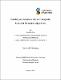## Stability and accuracy of electromagnetic transient simulation algorithms2020-12-01
Zhao, Huanfeng
##### Abstract
The main topic of this Ph.D. program is focus on the numerical stability and accuracy of Electromagnetic transient simulations. In the first part of this thesis, novel techniques are developed to evaluate the stability. Firstly, in order to adapt the existing stability analysis techniques for analyzing the stability of companion circuit based electromagnetic transient simulation, we prove the equivalency of state space equations models and electromagnetic companion circuit methods. Therefore, the stability conclusion for state space equations based simulation could be directly used for companion circuit model based simulation. After that, a novel technique is developed by applying Common Quadratic Lyapunov Function theory to analysis the numerical stability of lumped strictly passive switched system simulations. Based on the proposed method, it allows us to determine the stability of numerical algorithms in lumped strictly passive switched system simulations. This method is then extended to analyze the stability of simulations that use CDA or interpolation which are widely used approaches in modern simulators. In the second part, we developed a novel technique to assess the accuracy of electro-magnetic transient (EMT) simulations. The proposed method globally quantifies the simulation accuracy and is convenient to apply to large systems without explicitly forming the state space equations. It also permits the accuracy analysis of networks with distributed component such as frequency dependent transmission lines.
##### Keywords
Stability; Accuracy; Equivalency; Switched system;